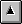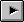8. The optional Double-Number word set

See: A.8 The optional Double-Number word set

8.1 Introduction

Sixteen-bit Forth systems often use double-length numbers. However, many Forths on small embedded systems do not, and many users of Forth on systems with a cell size of 32 bits or more find that the use of double-length numbers is much diminished. Therefore, the words that manipulate double-length entities have been placed in this optional word set.

None.

8.3.1 Environmental queries

Append table 8.1 to table 3.5.

See: 3.2.6 Environmental queries

Table 8.1 - Environmental Query Strings

String         Value data type   Constant?   Meaning
------         ---------------   ---------   -------
DOUBLE          flag             no          double-number word set present
DOUBLE-EXT      flag             no          double-number extensions word set present

8.3.2 Text interpreter input number conversion

When the text interpreter processes a number that is immediately followed by a decimal point and is not found as a definition name, the text interpreter shall convert it to a double-cell number.

For example, entering DECIMAL 1234 leaves the single-cell number 1234 on the stack, and entering DECIMAL 1234. leaves the double-cell number 1234 0 on the stack.

See: 3.4.1.3 Text interpreter input number conversion, RFI 0004 Number Conversion.

8.5 Compliance and labeling

8.5.1 ANS Forth systems

The phrase Providing the Double-Number word set shall be appended to the label of any Standard System that provides all of the Double-Number word set.

The phrase Providing name(s) from the Double-Number Extensions word set shall be appended to the label of any Standard System that provides portions of the Double-Number Extensions word set.

The phrase Providing the Double-Number Extensions word set shall be appended to the label of any Standard System that provides all of the Double-Number and Double-Number Extensions word sets.

8.5.2 ANS Forth programs

The phrase Requiring the Double-Number word set shall be appended to the label of Standard Programs that require the system to provide the Double-Number word set.

The phrase Requiring name(s) from the Double-Number Extensions word set shall be appended to the label of Standard Programs that require the system to provide portions of the Double-Number Extensions word set.

The phrase Requiring the Double-Number Extensions word set shall be appended to the label of Standard Programs that require the system to provide all of the Double-Number and Double-Number Extensions word sets.

8.6 Glossary

8.6.1 Double-Number words

8.6.1.0360 2CONSTANT
two-constant DOUBLE
( x1 x2 "<spaces>name" -- )

Skip leading space delimiters. Parse name delimited by a space. Create a definition for name with the execution semantics defined below.

name is referred to as a two-constant.

name Execution: ( -- x1 x2 )

Place cell pair x1 x2 on the stack.

See: 3.4.1 Parsing, A.8.6.1.0360 2CONSTANT

8.6.1.0390 2LITERAL
two-literal DOUBLE

Interpretation: Interpretation semantics for this word are undefined.

Compilation: ( x1 x2 -- )

Append the run-time semantics below to the current definition.

Run-time: ( -- x1 x2 )

Place cell pair x1 x2 on the stack.

8.6.1.0440 2VARIABLE
two-variable DOUBLE
( "<spaces>name" -- )

Skip leading space delimiters. Parse name delimited by a space. Create a definition for name with the execution semantics defined below. Reserve two consecutive cells of data space.

name is referred to as a two-variable.

name Execution: ( -- a-addr )

a-addr is the address of the first (lowest address) cell of two consecutive cells in data space reserved by 2VARIABLE when it defined name. A program is responsible for initializing the contents.

See: 3.4.1 Parsing, 6.1.2410 VARIABLE , A.8.6.1.0440 2VARIABLE

8.6.1.1040 D+
d-plus DOUBLE
( d1|ud1 d2|ud2 -- d3|ud3 )

Add d2|ud2 to d1|ud1, giving the sum d3|ud3.

8.6.1.1050 D-
d-minus DOUBLE
( d1|ud1 d2|ud2 -- d3|ud3 )

Subtract d2|ud2 from d1|ud1, giving the difference d3|ud3.

8.6.1.1060 D.
d-dot DOUBLE
( d -- )

Display d in free field format.

8.6.1.1070 D.R
d-dot-r DOUBLE
( d n -- )

Display d right aligned in a field n characters wide. If the number of characters required to display d is greater than n, all digits are displayed with no leading spaces in a field as wide as necessary.

See: A.8.6.1.1070 D.R

8.6.1.1075 D0<
d-zero-less DOUBLE
( d -- flag )

flag is true if and only if d is less than zero.

8.6.1.1080 D0=
d-zero-equals DOUBLE
( xd -- flag )

flag is true if and only if xd is equal to zero.

8.6.1.1090 D2*
d-two-star DOUBLE
( xd1 -- xd2 )

xd2 is the result of shifting xd1 one bit toward the most-significant bit, filling the vacated least-significant bit with zero.

See: A.8.6.1.1090 D2*

8.6.1.1100 D2/
d-two-slash DOUBLE
( xd1 -- xd2 )

xd2 is the result of shifting xd1 one bit toward the least-significant bit, leaving the most-significant bit unchanged.

See: A.8.6.1.1100 D2/

8.6.1.1110 D<
d-less-than DOUBLE
( d1 d2 -- flag )

flag is true if and only if d1 is less than d2.

8.6.1.1120 D=
d-equals DOUBLE
( xd1 xd2 -- flag )

flag is true if and only if xd1 is bit-for-bit the same as xd2.

8.6.1.1140 D>S
d-to-s DOUBLE
( d -- n )

n is the equivalent of d. An ambiguous condition exists if d lies outside the range of a signed single-cell number.

See: A.8.6.1.1140 D>S

8.6.1.1160 DABS
d-abs DOUBLE
( d -- ud )

ud is the absolute value of d.

8.6.1.1210 DMAX
d-max DOUBLE
( d1 d2 -- d3 )

d3 is the greater of d1 and d2.

8.6.1.1220 DMIN
d-min DOUBLE
( d1 d2 -- d3 )

d3 is the lesser of d1 and d2.

8.6.1.1230 DNEGATE
d-negate DOUBLE
( d1 -- d2 )

d2 is the negation of d1.

8.6.1.1820 M*/
m-star-slash DOUBLE
( d1 n1 +n2 -- d2 )

Multiply d1 by n1 producing the triple-cell intermediate result t. Divide t by +n2 giving the double-cell quotient d2. An ambiguous condition exists if +n2 is zero or negative, or the quotient lies outside of the range of a double-precision signed integer.

See: A.8.6.1.1820 M*/

8.6.1.1830 M+
m-plus DOUBLE
( d1|ud1 n -- d2|ud2 )

Add n to d1|ud1, giving the sum d2|ud2.

See: A.8.6.1.1830 M+

8.6.2 Double-Number extension words

8.6.2.0420 2ROT
two-rote DOUBLE EXT
( x1 x2 x3 x4 x5 x6 -- x3 x4 x5 x6 x1 x2 )

Rotate the top three cell pairs on the stack bringing cell pair x1 x2 to the top of the stack.

8.6.2.1270 DU<
d-u-less DOUBLE EXT
( ud1 ud2 -- flag )

flag is true if and only if ud1 is less than ud2.Table of ContentsNext Section